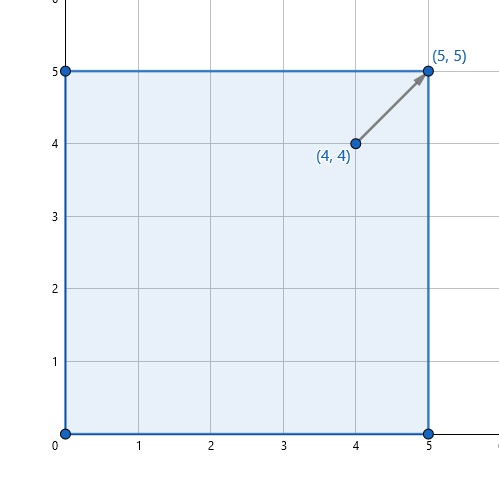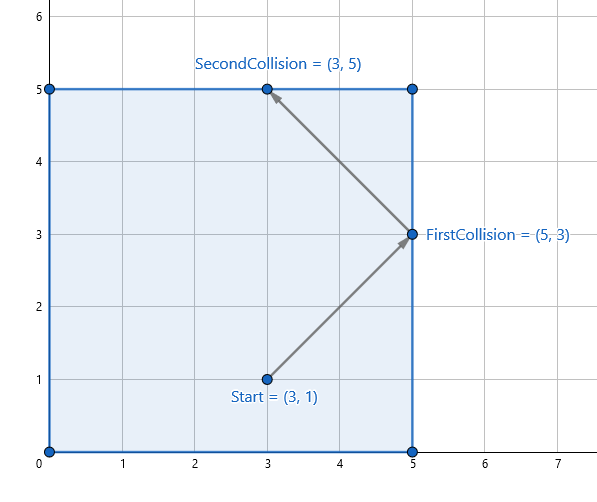## Point Of Impact Codechef January 2021 solution || Point Of Impact codechef january 2021 editorial -

### Problem statement-

You are playing a Billiards-like game on an $N×N$ table, which has its four corners at the points $\left\{\left(0,0\right),\left(0,N\right),\left(N,0\right),$ and $\left(N,N\right)\right\}$. You start from a coordinate $\left(x,y\right)$$\left(0 and shoot the ball at an angle ${45}^{\circ }$ with the horizontal. On hitting the sides, the ball continues to move with the same velocity and ensuring that the angle of incidence is equal to the angle of reflection with the normal, i.e, it is reflected with zero frictional loss. On hitting either of the four corners, the ball stops there and doesn’t move any further.

Find the coordinates of the point of collision, when the ball hits the sides for the ${K}^{th}$ time. If the ball stops before hitting the sides $K$ times, find the coordinates of the corner point where the ball stopped instead.

### Input:

• The first line of the input contains an integer $T$, the number of testcases.
• Each testcase contains a single line of input, which has four space separated integers - $N$$K$$x$$y$, denoting the size of the board, the number of collisions to report the answer for, and the starting coordinates.

### Output:

For each testcase, print the coordinates of the ball when it hits the sides for the ${K}^{th}$ time, or the coordinates of the corner point if it stopped earlier.

### Constraints

• $1\le T\le {10}^{5}$
• $2\le N\le {10}^{9}$
• $1\le K\le {10}^{9}$

• $30$ points : Sum of $K$ over all test cases $\le {10}^{7}$
• $70$ points : Original constraints.

### Sample Input:

2
5 5 4 4
5 2 3 1


### Sample Output:

5 5
3 5


### Explanation:

• Sample Case $1$ :

We are given a $5$ by $5$ board. We shoot the ball from coordinates $\left(4,4\right)$, and we need to find its coordinates after it has collided with sides $5$ times. However, after shooting, the ball goes directly to the corner $\left(5,5\right)$, and stops there. So we report the coordinates $\left(5,5\right)$.• Sample Case $2$ :

We are given a $5$ by $5$ board. We shoot the ball from the coordinates $\left(3,1\right)$, and we need to find its coordinates after it has collided with the sides twice. After shooting, it first hits the right side at $\left(5,3\right)$, and then the top side at $\left(3,5\right)$. So, we report $\left(3,5\right)$.Solution -

Actually this is a simple problem you need little bit of maths knowledge to solve this problem.
You have to find 4 points on where the ball is striking. the fact is that the ball can strike a maximum of 4 different point and after first 4 point  point of impact will start repeating.

You need to find 4 points of impact and ball direction(clockwise / anticlockwise) .ans differ according to ball direction.
Here is my code. look it and check how i implemented this logic.

Join telegram channel for code and editorial.-https://t.me/competitiveProgrammingDiscussion